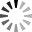# How to read Momentum and Rate of Change indicators to find buy and sell signals in uptrends and downtrends?

How to read Momentum and Rate of Change indicators to find buy and sell signals in uptrends and downtrends?M=P-Pn

Momentum and Rate of Change. It is an indicator that measures the current price change. With prices in the last N days.

provided that

• P = current closing price

• Pn = Closing price of n days before

And sometimes momentum may be measured by

R = P/Pn

which in the latter case We call it a measure of Rate of Change, which is another measure of change. Because in fact We can prove that R is equal to 1 + M/Pn, that is, if we subtract R by 1, we get the percentage change in price, while M is a measure of price change. In other words, R is a measure of percentage change. Conversion of price plus 1 itself (confused? ), so some traders subtract 1 from the formula to calculate Rate of Change to become R* = P/Pn - 1 as well. In this case, it will measure the Rate of Change around 0 instead of measuring around 1.

Some traders sometimes multiply R by 100 to get the actual percentage. Instead of the decimal point But everything is the same. In practice, Rate of Change (R) and Momentum (M) have very similar properties. And the readings are the same, only Momentum is measured from the zero line, but the Rate of Change is measured from the 1 line (or 100 if we are with 100).

Momentum lines built on small days Will be sensitive or susceptible to price changes. Than the line used longer time than which is smoother and less volatile If the price continues to move along the Uptrend and higher in the rate higher than the past, Momentum will continue to increase accordingly. But if the price increases at the same rate, the Momentum will move. In the side direction But if the price rises at a decreasing rate, momentum will weaken, so we can use momentum to “indicate price”. It is a measure of price acceleration and can guide prices.

• If the price is rising and Momentum crosses above the zero line and is moving up as well, it confirms Uptrend (use lines 1 or 100 instead in case of Rate of Change).

• If the price is going down, Momentum is falling and crosses the zero line as well. Will confirm Downtrend.

So a buy signal is generated when Momentum crosses the zero line and the price is in an uptrend. A sell signal is generated when Momentum crosses the zero line. And the price is in a Downtrend (likewise, use lines 1 or 100 instead of zero lines in case of Rate of Change)

An example of using momentum as an analytical tool. Can be considered from the example above The upper frame is the momentum, the bottom frame is the movement of the stock price and the moving average. The buy signals points appears is the point where the momentum is greater than 100 and is higher than the previous day. At the same time, the stock price is above the moving average as well (which should be significant. Or a better filter just comparing today's price with the previous day's price). On the other hand, it is where the momentum is less than 100 and lower than the day before. At the same time, the stock price is below the moving average, but the additional observation is that The profit received in each period will be more or less different. Because during period 1, there is quite an uptrend, there are a lot of selling signals points, observed from the 25-day ema line that has a positive slope. During the 2nd period, the slope of the 25-day ema line is less, but it is still uptrend. As for the 3rd period, the 25-day ema line is almost zero or fiat, so the profit during this period may not be worth it if considering the fees as well.

Теги:

 Спасибо:

Добавить файлы через драг-н-дроп, , или вставить из буфера обмена.